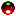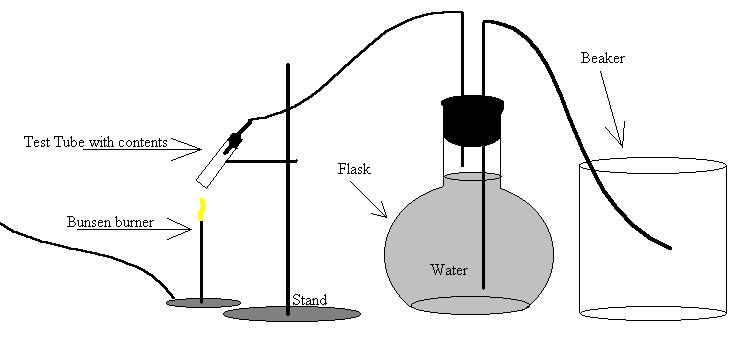CH - 120

Fall 2000

Molar Volume of Oxygen

Experiment #5

Submitted by:

Andrew J. Buettner

Partner:

none

Report Due: March 6, 2003Objective

The objective of this lab is to calculate the molar volume of oxygen.Apparatus

A) Equipment used

1) Florence flask

2) Pyrex test tube

3) Glass angle bends: 2

4) 2 holed rubber stopper

5) Single hole rubber stopper

6) Rubber tubing: 2 pieces

7) Stand

8) Bunsen burner

9) Graduated cylinder

10) Beaker

11) Potassium chlorate

B) DiagramMethod

1) Assemble the setup as shown in the diagram

2) Fill the flask with water

3) Ensure that the tubing is filled with water and has no air bubbles in it

4) Clamp off the end of the tubing to ensure that no air gets into the system

5) Mass the test tube alone

6) Add about 1g of potassium chlorate to the test tube

7) Mass the test tube and it's contents

8) Complete the setup and remove the clamp from the end of the tubing

9) Heat the test tube until the substance liquifies and re-solidifies

10) Ensure that all water is captured by the beaker

11) When reaction has completed and no further water is displaced, discontinue heating and clamp the end of the tubing

12) Measure the amount of water displaced using the graduated cylinderData

1) Initial mass of test tube: 31.677g

2) Mass of test tube with contents: 32.718g

3) Mass after burn: 32.270g

4) Volume of water displaced: 342.1ml

5) Temperature: 23OC

6) Barometric pressure: 774.5torrResults

1) Mass of oxygen released: .448g

2) Observed molar volume of oxygen: 24.4L/mole

3) Molar volume at STP: 22.9L/moleAnswers to Lab Questions

1) Q: If .10g KClO3 were heated to it's boiling point, 368OC, what volume of oxygen would be given off from the reaction given that the pressure was 1atm?

A: .0658L of oxygen would be given off

2) Q: When should the clamp come off of the rubber tubing, and when should it be on?

A: It should come off immediately after the heating process begins and be replaced soon after the heating process ends or when water stops coming out of the tube.

3) Q: The normal volume of oxygen per liter at STP is 22.392L/mole, what is the percent difference from this value?

A: There is a 2.27% error in the obtained values.

4) Q: If the Potassium chloride was not heated long enough to completely react, how would the molar volume of oxygen be affected?

A: It would have little or no effect on the outcome, provided that the amount that did react was captured accurately. This is true because both the volume of captured oxygen and the mass difference would be smaller proportionally.

5) Q: Using the data obtained from this lab, calculate R the ideal gas constant.

A: .0841 atm * L/ mole * OKConclusions

From this lab it is concluded that oxygen has a molar volume of 22.9L/mole. This is calculated experimentally with a percent error of 2.27% error from the given value of 2.932L/mole given by the ideal gas law.Attachments

1) Original Lab Data

2) Calculations